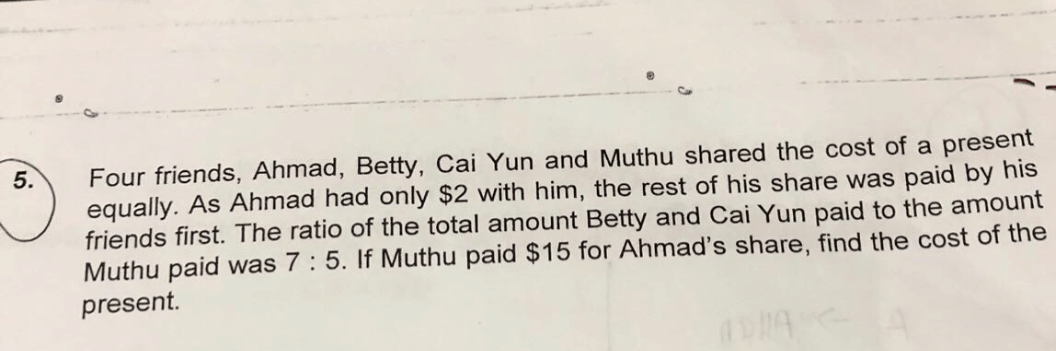# QuestionNeed help on this ratio question.

i assume the total cost of  present

=7u+5u+\$2

=12u+\$2

each person=(12u+\$2)/4  =3u+\$0.50

amt muthu help Ahmad to pay

= 5u-3u-\$0.50

=2u -\$0.50

2u-\$0.50=\$15

1u=7.75

12u =93

Is it correct

appreciate any help.

thanks

This is a Matrix question.

 Ahmad Betty Cai Yun Muthu Total Plan 5u – 15 5u – 15 5u – 15 5u – 15 20u – 60 Actual 17 7u 5u – 15 12u + 2

20u – 60 = 12u + 2

20u – 12u = 2 + 60

8u = 62

4u = 62 ÷ 2 = 31

12u = 31 × 3 = 93

93 + 2 = \$95

Appreciate the alternative answer

0 Replies 0 Likes

Yes, 12 units = \$93  is correct and the answer is \$93 + \$2 = \$95. In addition, I would advise you to add 2 \$ signs as shown below :

1u=\$7.75
12u =\$9.

1 Reply 0 Likes

Thanks . Noted my mistake

1 Reply 0 Likes

You are welcome. No worry. We are all learning each and every day. 🙂

0 Replies 0 Likes